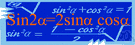# equation

(redirected from Axioms of equation)
Also found in: Thesaurus, Medical, Financial, Encyclopedia.

## e·qua·tion

(ĭ-kwā′zhən, -shən)
n.
1. The act or process of equating or of being equated.
2. The state of being equal.
3. Mathematics A statement asserting the equality of two expressions, usually written as a linear array of symbols that are separated into left and right sides and joined by an equal sign.
4. Chemistry A representation of a chemical reaction, usually written as a linear array in which the symbols and quantities of the reactants are separated from those of the products by an arrow or a set of opposing arrows.
5. A complex of variable elements or factors: "The world was full of equations ... there must be an answer for everything, if only you knew how to set forth the questions" (Anne Tyler).

## equation

(ɪˈkweɪʒən; -ʃən)
n
1. (Mathematics) a mathematical statement that two expressions are equal: it is either an identity in which the variables can assume any value, or a conditional equation in which the variables have only certain values (roots)
2. the act of regarding as equal; equating
3. the act of making equal or balanced; equalization
4. a situation, esp one regarded as having a number of conflicting elements: what you want doesn't come into the equation.
5. the state of being equal, equivalent, or equally balanced
6. a situation or problem in which a number of factors need to be considered
7. (Chemistry) See chemical equation
8. astronomy See personal equation

## e•qua•tion

(ɪˈkweɪ ʒən, -ʃən)

n.
1. the act of equating or making equal.
2. the state of being equated or equal.
3. equally balanced state; equilibrium.
4. an expression or a proposition, often algebraic, asserting the equality of two quantities.
5. a symbolic representation in chemistry showing the kind and amount of the starting materials and products of a reaction.
[1350–1400; Middle English < Latin]

## e·qua·tion

(ĭ-kwā′zhən)
1. Mathematics A written statement indicating the equality of two expressions. It consists of a sequence of symbols that is split into left and right sides joined by an equal sign. For example, 2 + 3 + 5 = 10 is an equation.
2. Chemistry A written representation of a chemical reaction, in which the symbols and amounts of the reactants are separated from those of the products by an equal sign, arrow, or a set of opposing arrows. For example, NaOH + HCl = NaCl + H2O is an equation.
ThesaurusAntonymsRelated WordsSynonymsLegend:
 Noun 1equation - a mathematical statement that two expressions are equalmath, mathematics, maths - a science (or group of related sciences) dealing with the logic of quantity and shape and arrangementregression equation, regression of y on x - the equation representing the relation between selected values of one variable (x) and observed values of the other (y); it permits the prediction of the most probable values of ylinear equation - a polynomial equation of the first degreequadratic, quadratic equation - an equation in which the highest power of an unknown quantity is a squarebiquadratic equation, biquadratic - an equation of the fourth degreedifferential equation - an equation containing differentials of a functionsimultaneous equations - a set of equations in two or more variables for which there are values that can satisfy all the equations simultaneouslymathematical statement - a statement of a mathematical relationexponential equation - an equation involving exponential functions of a variable 2 equation - a state of being essentially equal or equivalent; equally balanced; "on a par with the best"status, position - the relative position or standing of things or especially persons in a society; "he had the status of a minor"; "the novel attained the status of a classic"; "atheists do not enjoy a favorable position in American life"egalite, egality - social and political equality; "egality represents an extreme leveling of society"tie - equality of score in a contest 3 equation - the act of regarding as equalequatingequalisation, equalization, leveling - the act of making equal or uniform

## equation

noun the equation between higher spending and higher taxes

## equation

noun
The state of being equivalent:
Translations
مُعادَلةمُعادَلَه
rovnice
ligningregnestykke
yhtälö
egyenletkiegyenlítés
jafnaefnajafna

방정식
rovnica
enačba
ekvation
สมการ
phương trình

## equation

[ɪˈkweɪʒən] N
1. (Math) →
to enter (into) the equation (fig) →
fairness did not seem to enter into the equation
2. (= linking) the equation of sth with sthla identificación de algo con algo

[ɪˈkweɪʒən] n

## equation

n (Math, fig) → Gleichung f; the equation of religion and moralitydie Gleichsetzung von Religion mit Moralität; to balance the equation between work and leisure; that doesn’t even enter the equation

## equation

[ɪˈkweɪʒn] n (Math) →
equations of motion (Phys) →

## equate

(iˈkweit) verb
to regard as the same in some way. He equates money with happiness.
eˈquation (-ʒən) noun
1. a statement that two things are equal or the same. xy+xy=2xy is an equation.
2. a formula expressing the action of certain substances on others. 2H2 + O2 = 2 H2O is an equation.

## equation

rovnice ligning yhtälö jednadžba 等しくすること 방정식 ekvation สมการ phương trình
Site: Follow: Share:
Open / Close